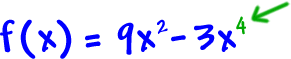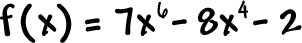This guy is a fifth degree polynomial:*The highest power on these wins!

Here's a fourth degree polynomial:So, what's the degree on this guy?It's reallySo, it's a first degree polynomial.

Here's a tricky one:Hmm...  there's NO x guy at all...  that's ZERO x guys...  So, it's a zero degree polynomial!

You can also write it likeTRY IT:

What are the degrees of these polynomials?